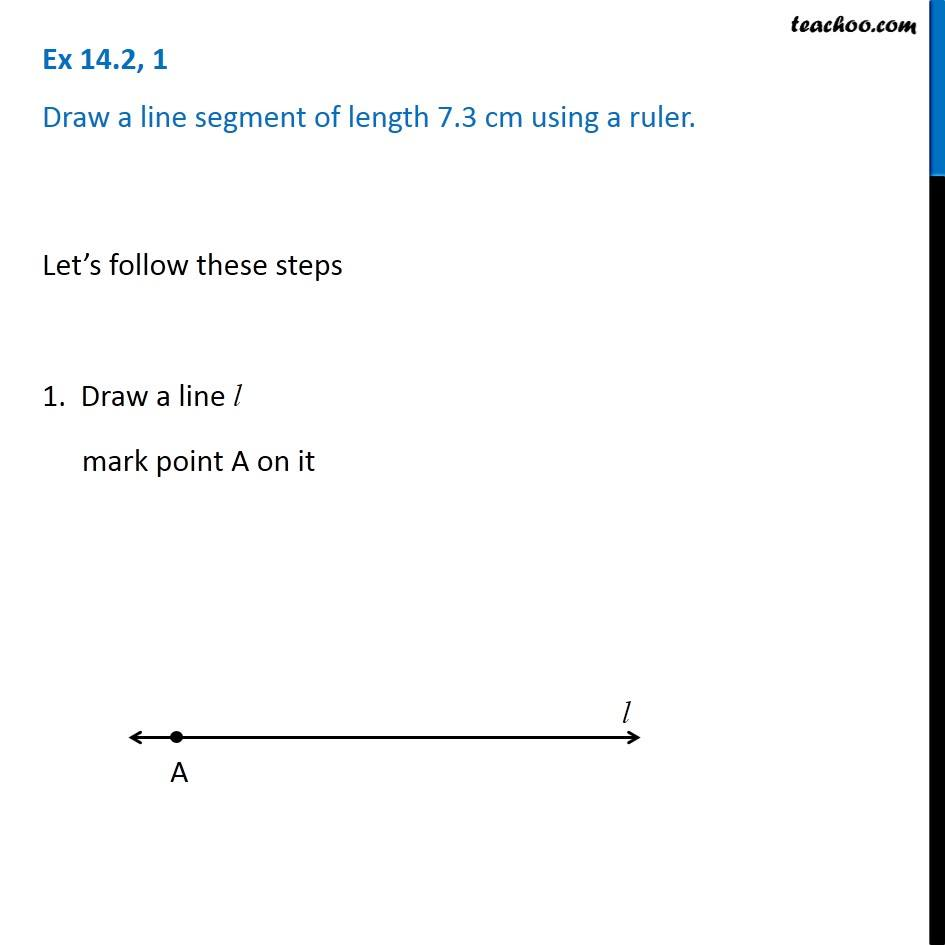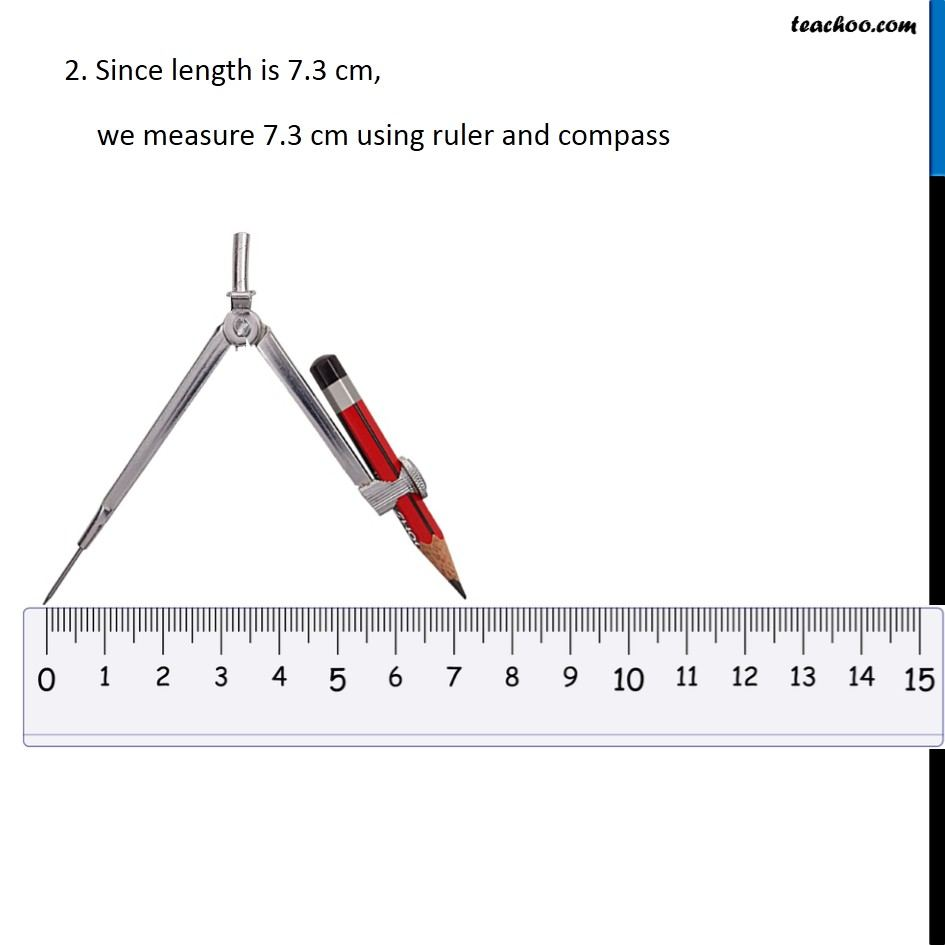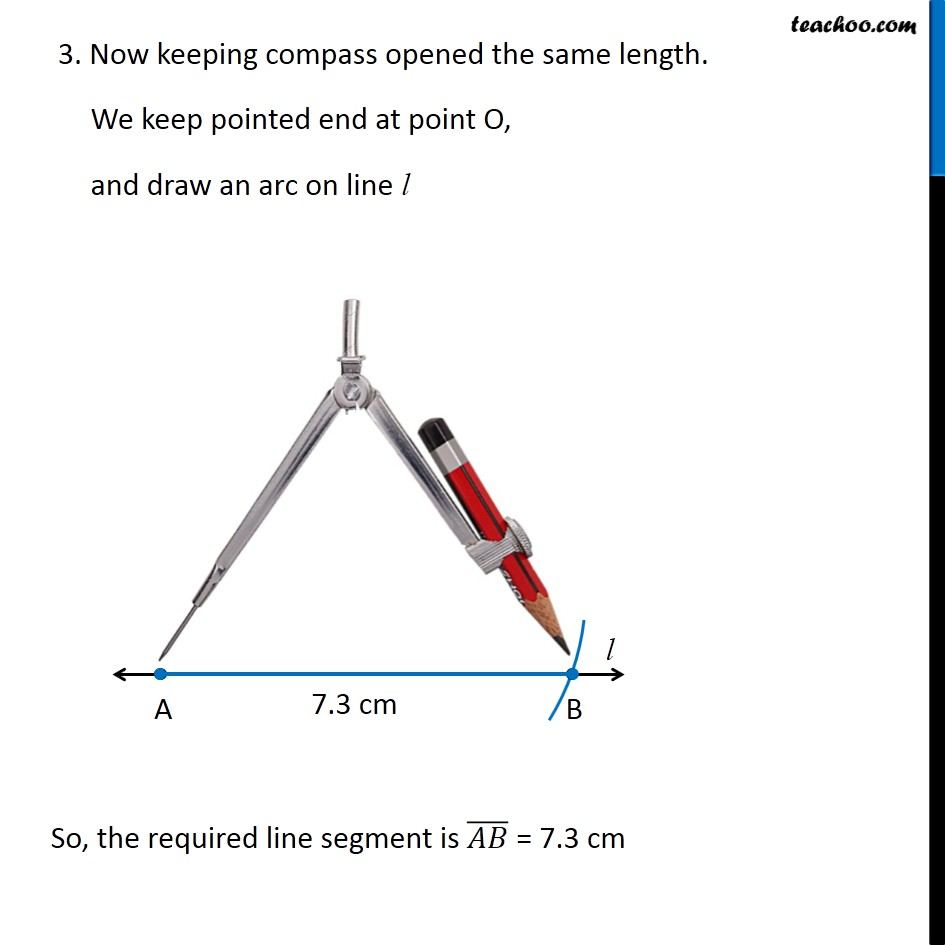1. Chapter 14 Class 6 Practical Geometry
2. Serial order wise
3. Ex 14.2

Transcript

Ex 14.2, 1 Draw a line segment of length 7.3 cm using a ruler. Let’s follow these steps 1. Draw a line l mark point A on it 2. Since length is 7.3 cm, we measure 7.3 cm using ruler and compass 3. Now keeping compass opened the same length. We keep pointed end at point O, and draw an arc on line l So, the required line segment is (𝐴𝐵) ̅ = 7.3 cm